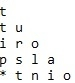# C Program for Reversed String Pattern

Given a string str, our task is to print its reversed pattern. The Pattern will be incremental in reversed order, and when the string is completed fill ‘*’ in the remaining places.

Like we enter a string “abcd”, now in first line we have to print “a” then in next line we have to print “cb” and then in third line we will print “**d”.

## Example

Input: str[] = { “abcd” }
Output: a
c b
* * d

Explanation

• In first line print 1 character
• In second line print 2 characters in reverse order
• In third line Print 3 characters in reverse order, if the string is less than 3 then print the characters and fill the blank spaces with *.
Input: str[] = {“tutorialspoint”}
Output:The approach used below is as follows

• We will traverse the string from i=0 and will check i<n && k<(n-i)*2 is true
• Then we will take a variable k and Set k as ((i*(i+1))/2)-1
• We will check If k >= n-1 then, will Print "* " else will print the string’s value in reverse order

## Algorithm

Start
In function int reverse_it(char str[], int n)
Step 1-> Declare and Initialize i, j=0 , k=0
Step 2-> Loop For i=0 and i<n && k<(n-i)*2 and i++
Set k as ((i*(i+1))/2)-1
Loop For j=0 and j<i && k<(n-i)*2 and j++
If k >= n-1 then,
Print "* "
Else
Print "%c ",str[k]
Decrement k by 1
End loop
Print new line
End loop
In Function int main(int argc, char const *argv[])
Step 1-> Declare and initialize string str[]
Step 2-> Declare and Initialize size as sizeof(str)/sizeof(str)
Step 3-> Call function reverse_it(str, size);
Stop

## Example

Live Demo

#include <stdio.h>
int reverse_it(char str[], int n) {
int i, j=0 , k=0;
for(i=0; i<n && k<(n-i)*2; i++) {
//Assigning k
k = ((i*(i+1))/2)-1;
for(j=0; j<i && k<(n-i)*2; j++) {
//will check if k is greater than the total number of characters
//then we will print * for filling the extra characters
if(k >= n-1)
printf("* ");
//print the string in reverse order
else
printf("%c ",str[k]);
k--;
}
//for line break after reverse sequence
printf("");
}
return 0;
}
//Main Function
int main(int argc, char const *argv[]) {
char str[] = {"tutorialspoint"};
int size = sizeof(str)/sizeof(str);
reverse_it(str, size);
return 0;
}

## Output

If run the above code it will generate the following output −